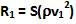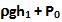Friday, May 29, 2009

Irodov Problem 1.326Suppose that v1 and v2 are the speeds with which the water flows out of the lower and the higher hole respectively.
The reactions exerted by the water flowing out by these two escaping jets is given byand. The net reaction on the container is given by,Suppose that the depth of the lower hole is h1. The velocity of water just before the entrance of the hole is assumed to be 0. The fluid pressure here is, where Po is the atmospheric pressure. Just outside the hole, the water escapes into open atmosphere i.e. into a region of atmospheric pressure. Applying Bernoulli's equation to points just before and after the hole we have,